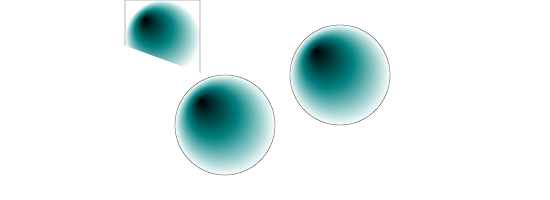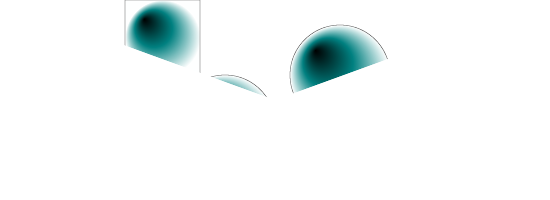# SVGBasics

## Where to Paint - Clipping

### Clipping

Clipping simply refers to cutting off the edges of an image that doesn't fit inside a given area. The bounding area can be simple, like a rectangle or a complex path. SVG allows you to apply a custom clipping path to drawing elements. The clipping path in the two examples below is an inverted triangle.

### Clipping Example

In this example, only one shape has a clipping path applied.<?xml version="1.0" standalone="no"?>
<!DOCTYPE svg PUBLIC "-//W3C//DTD SVG 1.1//EN" "http://www.w3.org/Graphics/SVG/1.1/DTD/svg11.dtd">

<svg viewBox = "0 0 1100 400" version = "1.1">
<desc>
</desc>
<defs>
<rect id = "r1" width = "150" height = "150" stroke = "black" stroke-width = "1"/>
<circle id = "r2" cx = "100" cy = "100" r = "100" stroke = "black" stroke-width = "1"/>
<circle id = "r3" cx = "100" cy = "100" r = "100" stroke = "black" stroke-width = "1"/>
<radialGradient id = "g1" cx = "50%" cy = "50%" r = "50%" fx = "25%" fy = "25%">
<stop stop-color = "black" offset = "0%"/>
<stop stop-color = "teal" offset = "50%"/>
<stop stop-color = "white" offset = "100%"/>
<clipPath id = "clip1">
<path d = "M 0 0 L 550 200 L 1100 0"/>
</clipPath>
</defs>
<g>
<use x = "250" y = "0" xlink:href = "#r1" fill = "url(#g1)" clip-path = "url(#clip1)"/>
<use x = "350" y = "150" xlink:href = "#r2" fill = "url(#g1)"/>
<use x = "580" y = "50" xlink:href = "#r3" fill = "url(#g1)"/>
</g>
</svg>

Here the same path is applied to all shapes by setting the clip-path attribute of the g container element. Note that the path describes a "V" shape.<?xml version="1.0" standalone="no"?>
<!DOCTYPE svg PUBLIC "-//W3C//DTD SVG 1.1//EN" "http://www.w3.org/Graphics/SVG/1.1/DTD/svg11.dtd">

<svg viewBox = "0 0 1100 400" version = "1.1">
<defs>
<rect id = "r1" width = "150" height = "150" stroke = "black" stroke-width = "1"/>
<circle id = "r2" cx = "100" cy = "100" r = "100" stroke = "black" stroke-width = "1"/>
<circle id = "r3" cx = "100" cy = "100" r = "100" stroke = "black" stroke-width = "1"/>
<radialGradient id = "g1" cx = "50%" cy = "50%" r = "50%" fx = "25%" fy = "25%">
<stop stop-color = "black" offset = "0%"/>
<stop stop-color = "teal" offset = "50%"/>
<stop stop-color = "white" offset = "100%"/>